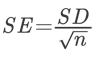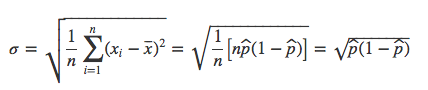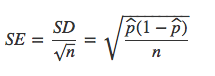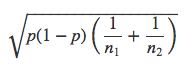Search
•Admin

# Standard Deviation & Standard Error

Updated: Jan 2, 2018# Standard Deviation

Standard Deviation is a measure of spread. A low standard deviation tell us the data is closely clustered around the mean while a high standard deviation says the data is dispersed over a wider range of values.

Standard deviation is often used when a data is approximately normally distributed. It isusually visualized to show how a data point is above or below the population mean. A data point that is beyond a certain number of standard deviations represents an outcome that is significantly below or above the average. This can be used to determine whether a result is “statistically significant” or part of “expected variation.”

In order to calculate the standard deviation, we measure the distance from the average for each element in a population and take the square of the difference. Then we divide it by the number of elements. This value gives us what is called the variance, which it's squareroot gives us the standard deviation:# Standard Error

In an AB test, our primary goal is to understand whether the proportions (conversion rates, add to carts, signup rates etc.) are statistically different enough between two treatments. So essentially, we are interested in how precisely we have measured the difference of proportions between the two groups. This measure is called the standard error of the difference. You can think of SE as the SD of the mean.

The formula for it is:The formula can be derived from the variance of a sum of independent random variables:## Standard Error for Proportions

For proportions, the Standard Error has a simplified version.

Let’s suppose that there are m 1s and (n-m) 0s among n subjects (1s can be customers that converted and 0s customers that didn't). Then we can say that (xi−x̄) will be equal to (1-m/n) for m observations and 0-m/n for (n-m) observations:Remember the standard deviation formula iswhich for proportions becomes:And using the formula for SE, we get SE for proportions as:## Standard Error of Difference

The difference of two normally distributed variates is also normally distributed, and the Standard Error of their difference is the square root of the sum of squares of their SEs :Proof can be found here: http://mathworld.wolfram.com/NormalDifferenceDistribution.html

For proportions this simply becomes:## Standard Error of Difference used in the “Two Proportions Z-Test”

Remember that we defined the Z-Score for the difference in proportions as:So essentially, in testing the difference of two population proportions, we have a test statistic that is of the form:

Proportion Differences / SE of Proportion Difference

Recall from above that the formula for SE of proportion differences is:So normally, we would do the above calculation to provide or best estimation towards the SE of proportion differences. Yet, since we know that A and B are two different samples from the same population, we have a really strong reason to beleive that their variances would be equal. Knowing that gives us a better accuracy in estimating the SE of the proportion differences. For that reason, we equate the variances of A and B via a common value of p, say, that is, p1=p2=p where we simply define p (the pooled proportion) as:where Y is the number of successes (conversions, add to carts etc.)

In that case, the SE of proportion differences actually becomes: